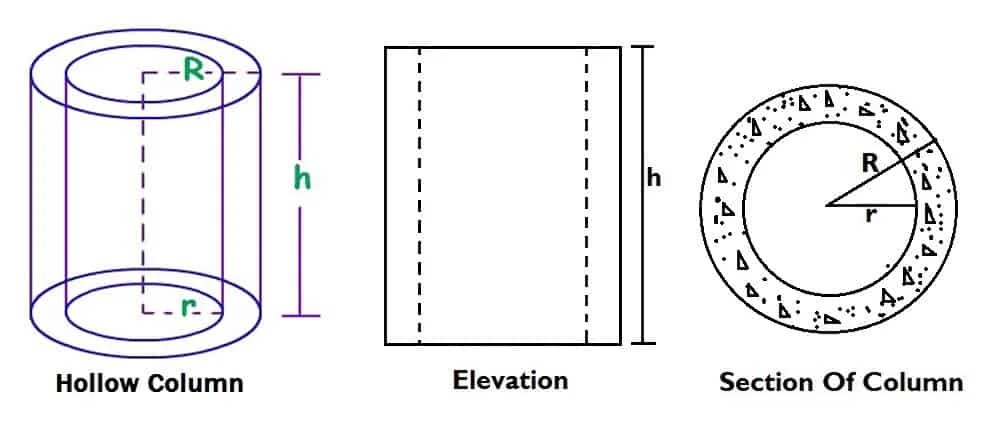## Register Now

Lorem ipsum dolor sit amet, consectetur adipiscing elit.Morbi adipiscing gravdio, sit amet suscipit risus ultrices eu.Fusce viverra neque at purus laoreet consequa.Vivamus vulputate posuere nisl quis consequat.

## How To Calculate Volume Of Hollow Column:Given,

h = 4 m.

R = 0.8 m.

r = 0.4 m.

Where

h = Height of the hollow column.

R = Centre to the outer radius of the hollow column.

r = Centre to the internal radius of the hollow column.

The formula for calculating the volume of hollow column is given below:

Volume = (πR²h – πr²h)

= πh ( R² – r²)

= 3.14 x 4 ( 0.8² – 0.4²) [∵ π =3.14]

= 12.56 ( 0.64 – 0.16)

= 12.56 x 0.48

= 6.028 m³.

## Comment ( 1 )

1. This is so helpful and I am learning so many things from here.

error: Content is protected !!